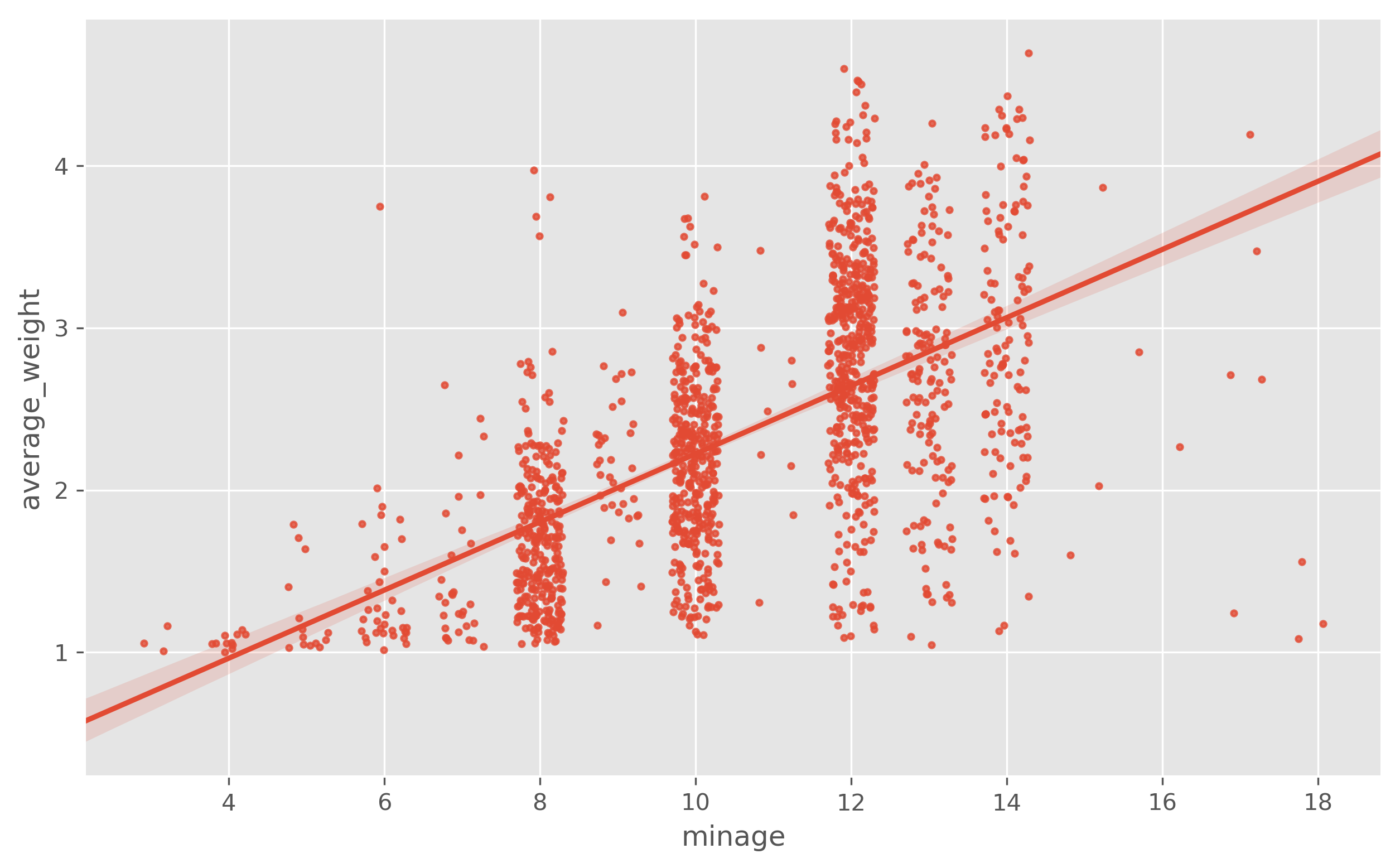In :
%pylab inline
import seaborn as sns
import pandas as pd
import statsmodels.api as sm

figsize(10,6)
plt.rcParams['figure.dpi'] = 300

plt.style.use('ggplot')
#plt.rcParams['font.family'] = 'Myriad Pro'
plt.rcParams['text.color'] = '#555555'

Populating the interactive namespace from numpy and matplotlib

C:\Users\Danny\Anaconda\lib\site-packages\statsmodels\compat\pandas.py:56: FutureWarning: The pandas.core.datetools module is deprecated and will be removed in a future version. Please use the pandas.tseries module instead.
from pandas.core import datetools

In :
# Load data

df = pd.read_csv('games_Jan2018.csv')
df.set_index('id', inplace=True)

df.type.value_counts()

# Let's filter out expansions, and focus our analysis on base games
df = df[df.type == 'boardgame']

# A little bit of data cleaning to set zeros to NAs where they should be NAs
df[['yearpublished', 'minplayers', 'maxplayers', 'playingtime', 'minplaytime', 'maxplaytime', 'minage']] = \
df[['yearpublished', 'minplayers', 'maxplayers', 'playingtime', 'minplaytime', 'maxplaytime', 'minage']].replace(0,pd.np.nan)

enough_ratings = df.users_rated >= 150
enough_weights = df.total_weights >= 150
enough_ratings.sum(), enough_weights.sum()

Out:
(6455, 1081)
In [ ]:


In :
plt.style.use('ggplot')
#plt.rcParams['font.family'] = 'Myriad Pro'
plt.rcParams['text.color'] = '#555555'


# Load data¶

In :
df = pd.read_csv('games_Jan2018.csv')
df.set_index('id', inplace=True)

In :
df.type.value_counts()

Out:
boardgame             80105
boardgameexpansion    15672
Name: type, dtype: int64
In :
# Let's filter out expansions, and focus our analysis on base games
df = df[df.type == 'boardgame']

In :
# A little bit of data cleaning to set zeros to NAs where they should be NAs
df[['yearpublished', 'minplayers', 'maxplayers', 'playingtime', 'minplaytime', 'maxplaytime', 'minage']] = \
df[['yearpublished', 'minplayers', 'maxplayers', 'playingtime', 'minplaytime', 'maxplaytime', 'minage']].replace(0,pd.np.nan)

In :
enough_ratings = df.users_rated >= 150
enough_weights = df.total_weights >= 150
enough_ratings.sum(), enough_weights.sum()

Out:
(6455, 1081)

# Complexity / weight¶

BGG allows users to rate the "weight" or complexity of a game. This is a single measure for each game from a scale from 1-5 and it doesn't decouple the different types of complexity (e.g. Chess that's relatively easy in terms of rules, but can be quite complex in terms of how to use those rules to play as opposed to Terra Mystica, where it take a while to learn all the rules, but once you've got the hang of the rules, the gameplay is relatively straight forward)

N.B. There's probably elegant terminology for these different types of complexity. Colm may know the answer.

Let's find out how reliable this information is by running some rodimentary tests against some simple intuition-based proxies for complexity

In :
# Testing weight rating against minimum age

sns.regplot(x='minage', y='average_weight', data=df[df.total_weights>100], x_jitter=.3, scatter_kws={'s':6})

Out:
<matplotlib.axes._subplots.AxesSubplot at 0x13ed2a90>Min age is a loose proxy for complexity, but it can also be filtered due to content type such as very explicit material e.g. Cards against Humanity)

In :
cax = sns.regplot(x='average_weight',
y='average_rating',
data=df[enough_weights],
scatter_kws={'alpha':0.8,
's':10,
'color':None,
'cmap':'seismic_r',},
line_kws={'lw':1,
'ls':':',
'color':'k'})

plt.gca().annotate('Monopoly', xy=(1.685, 4.42057), xytext=(1.5,3), arrowprops=dict(facecolor='black', arrowstyle="->",edgecolor='k'))
plt.gca().annotate("Sid Meier's Civilization: The Boardgame", xy=(3.6454, 5.59183), xytext=(3,4.5), arrowprops=dict(facecolor='black', arrowstyle="->",edgecolor='k'))
plt.gca().annotate("Risk", xy=(2.1072, 5.57929), xytext=(2,4), arrowprops=dict(facecolor='black', arrowstyle="->",edgecolor='k'))
plt.gca().annotate("Gloomhaven", xy=(3.78, 9.00657), xytext=(3,9.1), arrowprops=dict(facecolor='black', arrowstyle="->",edgecolor='k'))
plt.gca().annotate("Kingdom Death: Monster", xy=(4.186, 8.97231), xytext=(3.2,8.7), arrowprops=dict(facecolor='black', arrowstyle="->",edgecolor='k'))
plt.gca().annotate("Pandemic Legacy", xy=(2.8026, 8.66878), xytext=(2.2,9.0), arrowprops=dict(facecolor='black', arrowstyle="->",edgecolor='k'))
plt.gca().annotate("Codenames", xy=(1.3535, 7.90691), xytext=(1.0,8.5), arrowprops=dict(facecolor='black', arrowstyle="->",edgecolor='k'))
plt.gca().annotate("7 Wonders: Duel", xy=(2.2463, 8.19443), xytext=(1.5,9.1), arrowprops=dict(facecolor='black', arrowstyle="->",edgecolor='k'))
plt.gca().annotate("Patchwork", xy=(1.7131, 7.83136), xytext=(1.2,8.8), arrowprops=dict(facecolor='black', arrowstyle="->",edgecolor='k'))
plt.gca().annotate("Blackbeard", xy=(3.3218, 6.09174), xytext=(3,5), arrowprops=dict(facecolor='black', arrowstyle="->",edgecolor='k'))
plt.gca().annotate("Rise and Decline of the Third Reich", xy=(4.2945, 6.79503), xytext=(3.5,6), arrowprops=dict(facecolor='black', arrowstyle="->",edgecolor='k'))

plt.ylabel('Average rating')
plt.xlabel('Average weight score (complexity)')
plt.title('Rating vs complexity')

#ax1 = plt.gcf().add_axes([0.91, 0.125, 0.01, 0.75])
#norm = mpl.colors.Normalize(vmin=3, vmax=5)
#cb1 = mpl.colorbar.ColorbarBase(ax1, cmap='seismic_r',
#                                norm=norm,
#                                orientation='vertical')
#cb1.set_ticks([pd.np.arange(3,6,1)])
#cb1.set_ticklabels(['1,000','10,000','100,000'])
#cb1.set_label('Number of owners')

plt.gcf().set_size_inches(10,6)